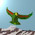## Saturday, May 8, 2010

### Top Down Numbers

From a PDG review:
The CKM elements $| V_{td} |$ and $| V_{ts} |$ cannot be measured from tree level decays of the top quark, so one has to rely on determinations from $B \overline{B}$ oscillations mediated by box diagrams or loop mediated rare K and B decays. Theoretical uncertainties in hadronic effects limit the accuracy of the current determinations.
The 2008 review suggests that $| V_{td} / V_{ts} | < 0.216$, and this bound is broken by the parameter fits (which used this data) in recent posts. Although this fit was rough, it yields a value of $0.242$, which is significantly higher than the Fermilab estimate. It is still within the other bounds given by $B$ and $K$ rates. Note, however, that the current best estimates for CKM parameters also puts $| V_{td} / V_{ts} |$ at too high a value, so perhaps one should not take this bound too seriously, since it relies on Standard Model calculations.

With this fit, the parameter $2 \beta_{s}$ comes out to be $0.03877$. This is in disagreement with our old rough (and strangely viable) value (Carl's circulant sum now gives a result of about $0.05$) but in mysteriously good agreement with the Standard Model fit for $2 \beta_{s}$.

Update: OK, so I have now checked the old estimate carefully. The difference between the $0.04$ and the larger value (roughly $0.9$) comes down to the signs used in the $(cb)$ term. The value $0.04$ is correct.

Case 1: The $0.04$ value comes from taking $0.2275 + 1.000809 i$ as the conjugate of the term in $M$ (unnormalised), which is the natural thing to do. In this case, the three angles for $(tb)$, $(cs)$ and $(cb)$ all cancel, and the $0.04$ basically comes from the phase for the $(ts)$ term, which is $0.019386$ radians.

Case 2: The larger value may be obtained from adjusting the phased CKM matrix so that the terms in the $2 \beta_s$ ratio have matching signs. That is, it takes $0.2275 - 1.000809 i$, in which case the $1.347278$ does not cancel and the $2 \beta_s$ value is now around $0.9$. This is the same as taking $V_{cb}$ instead of $V_{cb}^{*}$. I cannot see a good reason for doing this.

1.OK, I think Maxima is doing this right, and $0.04$ is the right answer. If you look at it by hand, the quotient of phases comes down to a number close to $\pi$. So it matters that we keep track of the $\pi$s when summing all the phases, and this is probably what we did wrong when we were doing it by hand.

2.P.S. I am not upset that we get the SM value, but this means that those experimentalists are going to have to get off their asses and sort this number out ...

3.For those who don't remember: the current estimates from CDF/D0 do not agree with this $0.04$ (although the SM must agree with this) but they do seem to agree with the supposedly bad (Carl's matrix has not changed) number that we calculated last year. That is, the difference between (i) the 'true' phase $0.04$ and (ii) the phase one gets by ignoring a bunch of signs is EXACTLY the discrepancy between (i) the SM fit and (ii) the current experimental range.

4.Just to clarify:

(1) the CKM parameters determine the relative weak force interaction strengths.

(2) the weak force interaction rate depends on the relative mass of the weak gauge boson to the particle being considered. Neglecting electroweak theory for a moment, in Fermi's theory of beta decay, the weak force has a strength equal to the electromagnetic force strength multiplied by the impressive looking ratio ratio: (π^2)h(M^4)/[T(c^2)m^5)] (reference: Matthews Quantum Mechanics textbook), where T is the effective lifetime of the particle (i.e. halflife times the factor 1/ln2 ~ 1.44), m is the mass of the emitted fermion and M is the mass of the particle before it decays.

(3) therefore, there seems to be a link between the CKM parameters and the masses of fundamental particles.

Do you agree? (Sorry for posting 2 comments in a row. Delete them if you like, I'll copy to my blog just in case.)

5.Nigel, you must stop putting big links on my blog, or I will ban you from the blog. I don't care what their content is. And of course I think the CKM parameters are related to masses ... duh. If you actually bothered reading anything I wrote you would understand that.

6.OK, Nigel, so if the masses are responsible for the sign flip mentioned in the update (which has nothing at all to do with factors of $\pi$) then it makes the phased CKM no longer the sum of two circulants. That is, we end up looking at a matrix with the same norm squares, but unequal row sums. It could be relevant, because the Fourier matrix (unlike $R_n$) is like this.

7." I don't care what their content is"

I know you don't care a damn about simple mechanisms, that's why I'm asking where you claim to get Pi from, and if it is related to the factors of Pi in the link you deleted. If you cared about being clear rather than just rude, you'd give an answer.

Nige

8."Nigel, you must stop putting big links on my blog, or I will ban you from the blog"

It was a small link. I will stop reading your blog now, so I won't make any more comments, go celebrate with more rudeness!

Nige